## Square Wave Generator Using Op amp:

The Square Wave Generator Using Op amp means the astable multivibrator circuit using op-amp, which generates the square wave of required frequency. The Fig. 2.83 shows the square wave generator using op amp.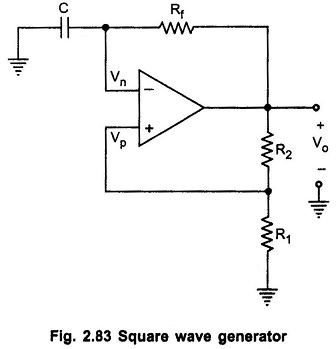It looks like a comparator with hysteresis (schmitt trigger), except that the input voltage is replaced by a capacitor. The circuit has a time dependent elements such as resistance and capacitor to set the frequency of oscillation.

As shown in the Fig. 2.83 the comparator and positive feedback resistors R1 and R2 form an inverting schmitt trigger.

When Vo  is at +Vsat, the feedback voltage is called the upper threshold voltage VUT and is given as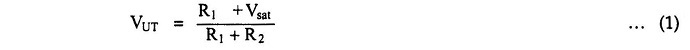When Vo is at -Vsat, the feedback voltage is called the lower-threshold voltage VLT and is given as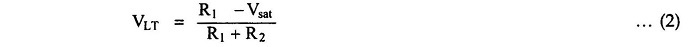When power is turn ON, Vo automatically swings either to +Vsat or to -Vsat since these are the only stable states allowed by the schmitt trigger. Assume it swings to +Vsat. With Vo = +Vsat we have – Vp = VUT and capacitor starts charging towards +Vsat through the feedback path provided by the resistor Rf to the inverting (-) input. This is illustrated in Fig. 2.84 (a). As long as the capacitor voltage VC is less than VUT, the output voltage remains at +Vsat.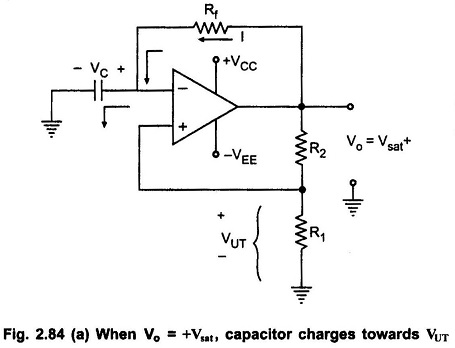As soon as VC charges to a value slightly greater than VUT, the (-) input goes positive with respect to the (+) input. This switches the output voltage from +Vsat to -Vsat and we have Vp = VLT , which is negative with respect to ground. As Vo switches to -Vsat, capacitor starts discharging via Rf, as shown in the Fig. 2.84 (b).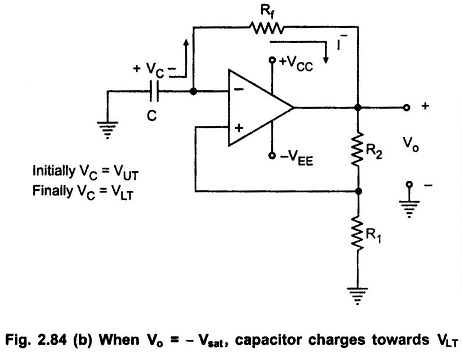The  current   I – discharges capacitor to 0 V and recharges capacitor to VLT. When VC becomes slightly more negative than the feedback voltage VLT, output voltage Vo switches back to +Vsat. As a result, the condition in Fig. 2.84(a) is reestablished except that capacitor now has a initial charge equal to VLT. The capacitor will discharge from VLT to 0V and then recharge to VUT, and the process is repeating. Once the initial cycle is completed, the waveform become periodic, as shown in the Fig. 2.84(c).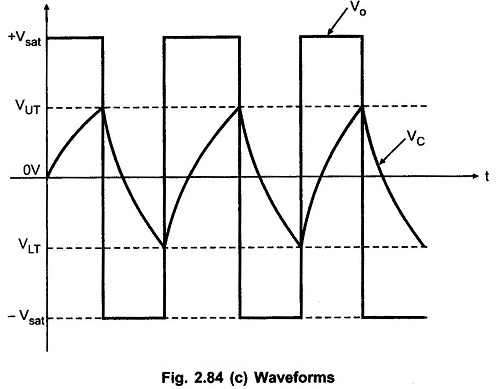### Frequency of Oscillation:

The frequency of oscillation of Square Wave Generator Using Op amp is determined by the time it takes the capacitor to charge from VUT to VLT and vice versa. The voltage across the capacitor as a function of time is given as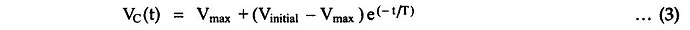where

• VC(t) is the instantaneous voltage across the capacitor.
• Vinitial is the initial voltage
• Vmax is the voltage toward which the capacitor is charging.

Let us consider the charging of capacitor from VLT to VUT, where VLT is the initial voltage, VUT is the instantaneous voltage and +Vsat is the maximum voltage. At t = T1, voltage across capacitor reaches VUT and therefore equation (3) becomes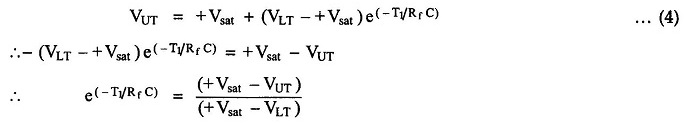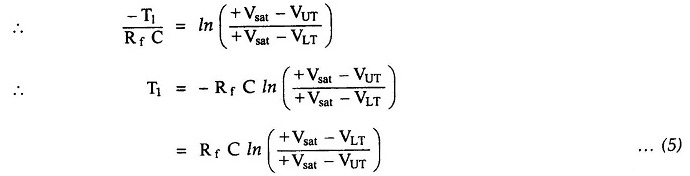The time taken by capacitor to charge from VUT to VLT is same as time required for charging capacitor from VLT to VUT. Therefore, total time required for one oscillation is given asThe frequency of oscillation can be determined as fo = 1/T, where T represents the time required for one oscillation.

Substituting the value of T we get,Scroll to Top